Updating search results...

# 194 Results

View
Selected filters:
• probability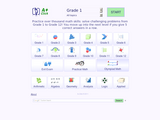Conditional Remix & Share Permitted
CC BY-NC-SA
Rating
0.0 stars

A+ Click is an interactive collection of more than 3700 math problems and answers for K-1 K-12 school program. It defines the personal level of math knowledge. You move up into the next level if you give 5 correct answers in a row. Practice makes perfect.

Subject:
Algebra
Geometry
Mathematics
Material Type:
Activity/Lab
Assessment
Game
Lecture Notes
Provider:
NSDL Staff
Provider Set:
Mathematics Gateways and Resources
Author:
Igor Kokcharov
02/16/2011Unrestricted Use
CC BY
Rating
0.0 stars

An introduction to probability through the example of flipping a quarter and rolling a die. it is a practice lesson.

Subject:
Applied Science
Arts and Humanities
Engineering
Material Type:
Lecture Notes
Author:
09/13/2020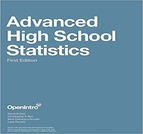Conditional Remix & Share Permitted
CC BY-SA
Rating
0.0 stars

This textbook is part of the OpenIntro Statistics series and offers complete coverage of the high school AP Statistics curriculum. Real data and plenty of inline examples and exercises make this an engaging and readable book. Links to lecture slides, video overviews, calculator tutorials, and video solutions to selected end of chapter exercises make this an ideal choice for any high school or Community College teacher. In fact, Portland Community College recently adopted this textbook for its Introductory Statistics course, and it estimates that this will save their students \$250,000 per year. Find out more at: openintro.org/ahss

View our video tutorials here:
openintro.org/casio
openintro.org/TI

Subject:
Mathematics
Statistics and Probability
Material Type:
Textbook
Provider:
OpenIntro
Author:
Christopher Barr
David Diez
Leah Dorazio
Mine Cetinkaya-Rundel
04/11/2018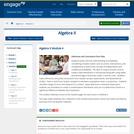Conditional Remix & Share Permitted
CC BY-NC-SA
Rating
0.0 stars

Students build a formal understanding of probability, considering complex events such as unions, intersections, and complements as well as the concept of independence and conditional probability.  The idea of using a smooth curve to model a data distribution is introduced along with using tables and technology to find areas under a normal curve.  Students make inferences and justify conclusions from sample surveys, experiments, and observational studies.  Data is used from random samples to estimate a population mean or proportion.  Students calculate margin of error and interpret it in context.  Given data from a statistical experiment, students use simulation to create a randomization distribution and use it to determine if there is a significant difference between two treatments.

Find the rest of the EngageNY Mathematics resources at https://archive.org/details/engageny-mathematics.

Subject:
Algebra
Mathematics
Material Type:
Module
Provider:
New York State Education Department
Provider Set:
EngageNY
03/24/2016Conditional Remix & Share Permitted
CC BY-NC-SA
Rating
0.0 stars

(Nota: Esta es una traducción de un recurso educativo abierto creado por el Departamento de Educación del Estado de Nueva York (NYSED) como parte del proyecto "EngageNY" en 2013. Aunque el recurso real fue traducido por personas, la siguiente descripción se tradujo del inglés original usando Google Translate para ayudar a los usuarios potenciales a decidir si se adapta a sus necesidades y puede contener errores gramaticales o lingüísticos. La descripción original en inglés también se proporciona a continuación.)

Los estudiantes crean una comprensión formal de la probabilidad, considerando eventos complejos como sindicatos, intersecciones y complementos, así como el concepto de independencia y probabilidad condicional. La idea de usar una curva suave para modelar una distribución de datos se introduce junto con el uso de tablas y tecnología para encontrar áreas bajo una curva normal. Los estudiantes hacen inferencias y justifican conclusiones de encuestas de muestra, experimentos y estudios de observación. Los datos se usan de muestras aleatorias para estimar una media o proporción de población. Los estudiantes calculan el margen de error y lo interpretan en contexto. Dados los datos de un experimento estadístico, los estudiantes usan la simulación para crear una distribución de aleatorización y lo usan para determinar si hay una diferencia significativa entre dos tratamientos.

Encuentre el resto de los recursos matemáticos de Engageny en https://archive.org/details/engageny-mathematics.

English Description:
Students build a formal understanding of probability, considering complex events such as unions, intersections, and complements as well as the concept of independence and conditional probability.  The idea of using a smooth curve to model a data distribution is introduced along with using tables and technology to find areas under a normal curve.  Students make inferences and justify conclusions from sample surveys, experiments, and observational studies.  Data is used from random samples to estimate a population mean or proportion.  Students calculate margin of error and interpret it in context.  Given data from a statistical experiment, students use simulation to create a randomization distribution and use it to determine if there is a significant difference between two treatments.

Find the rest of the EngageNY Mathematics resources at https://archive.org/details/engageny-mathematics.

Subject:
Algebra
Mathematics
Material Type:
Module
Provider:
New York State Education Department
Provider Set:
EngageNY
03/24/2016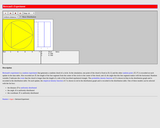Unrestricted Use
CC BY
Rating
0.0 stars

This resource consists of a Java applet and expository text. The applet is a simulation of Bertrand's experiment: a random chord on a circle The event of interest is whether the length of the chord is larger than the length of the inscribed equilateral triangle. Three models for generating the random chord can be used.

Subject:
Mathematics
Statistics and Probability
Material Type:
Activity/Lab
Interactive
Simulation
Provider:
University of Alabama in Huntsville
Provider Set:
Virtual Laboratories in Probability and Statistics
Author:
Kyle Siegrist
02/16/2011Unrestricted Use
CC BY
Rating
0.0 stars

This resource consists of a Java applet and expository text. The applet illustrates Bayesian estimation of the probability of heads for a coin. The prior beta distribution, true probability of heads, and the sample size can be specified. The applet shows the posterior beta distribution.

Subject:
Mathematics
Statistics and Probability
Material Type:
Activity/Lab
Interactive
Simulation
Provider:
University of Alabama in Huntsville
Provider Set:
Virtual Laboratories in Probability and Statistics
Author:
Kyle Siegrist
02/16/2011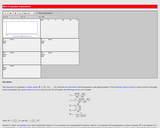Unrestricted Use
CC BY
Rating
0.0 stars

This resource consists of a Java applet and expository text. The applet simulates a random sample from a beta distribution, and computes standard point estimates of the left and right parameters. The bias and mean square error are also computed.

Subject:
Mathematics
Statistics and Probability
Material Type:
Activity/Lab
Interactive
Simulation
Provider:
University of Alabama in Huntsville
Provider Set:
Virtual Laboratories in Probability and Statistics
Author:
Kyle Siegrist
02/16/2011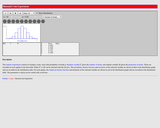Unrestricted Use
CC BY
Rating
0.0 stars

This resource consist of a Java applet and expository text. The applet simulates Bernoulli trials in terms of coin tosses. The random variables of interest are the number of heads and the proportion of heads. The number of coins and the probability of heads can be varied. The applet illustrates the law of large numbers and the central limit theorem.

Subject:
Mathematics
Statistics and Probability
Material Type:
Activity/Lab
Interactive
Simulation
Provider:
University of Alabama in Huntsville
Provider Set:
Virtual Laboratories in Probability and Statistics
Author:
Kyle Siegrist
02/16/2011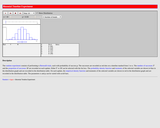Unrestricted Use
CC BY
Rating
0.0 stars

This resource consists of a Java applet and expository text. The applet simulates Bernoulli trials in terms of random points on a timeline. The random variables of interest are the number of successes and the proportion of successes. The number of trials and the probability of success can be varied. This applet illustrates the law of large numbers, the central limit theorem, and the binomial distribution.

Subject:
Mathematics
Statistics and Probability
Material Type:
Activity/Lab
Interactive
Simulation
Provider:
University of Alabama in Huntsville
Provider Set:
Virtual Laboratories in Probability and Statistics
Author:
Kyle Siegrist
02/16/2011Unrestricted Use
CC BY
Rating
0.0 stars

Biology is designed for multi-semester biology courses for science majors. It is grounded on an evolutionary basis and includes exciting features that highlight careers in the biological sciences and everyday applications of the concepts at hand. To meet the needs of today&rsquo;s instructors and students, some content has been strategically condensed while maintaining the overall scope and coverage of traditional texts for this course. Instructors can customize the book, adapting it to the approach that works best in their classroom. Biology also includes an innovative art program that incorporates critical thinking and clicker questions to help students understand&mdash;and apply&mdash;key concepts.

Subject:
Biology
Life Science
Material Type:
Full Course
Provider:
Rice University
Provider Set:
OpenStax College
08/22/2012Conditional Remix & Share Permitted
CC BY-NC
Rating
0.0 stars
Rating
0.0 stars
Subject:
Applied Science
Biology
Life Science
Material Type:
Module
07/10/2017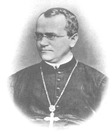Conditional Remix & Share Permitted
CC BY-NC
Rating
0.0 stars

By the end of this section, you will be able to:Describe the scientific reasons for the success of Mendel&rsquo;s experimental workDescribe the expected outcomes of monohybrid crosses involving dominant and recessive allelesApply the sum and product rules to calculate probabilities

Subject:
Applied Science
Biology
Life Science
Material Type:
Module
07/10/2017Only Sharing Permitted
CC BY-NC-ND
Rating
0.0 stars

A free online textbook for biophysical chemistry. The book covers probability, statistics, thermodynamics, kinetics, Monte Carlo methods, biochemistry, diffusion, stochastic processes, and others.

Subject:
Biology
Chemistry
Life Science
Physical Science
Physics
Material Type:
Textbook
Author:
John Della Rosa
10/05/2023Some Rights Reserved
Rating
0.0 stars

Try this fun problem! In any group of six people, what is the probability that everyone was born in different months?

Subject:
Education
Mathematics
Material Type:
Diagram/Illustration
Lecture Notes
Provider:
SMARTR
Provider Set:
SMARTR: Virtual Learning Experiences for Youth
Author:
KnowNet Construction, Inc.
11/06/2004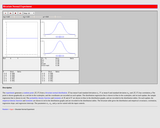Unrestricted Use
CC BY
Rating
0.0 stars

This resource consists of a Java applet and expository text. The applet simulates the bivariate normal distribution. The means are set at 0, but the standard deviations and the correlation can be varied. Simulated points from the distribution are shown as dots in a scatterplot.

Subject:
Mathematics
Statistics and Probability
Material Type:
Activity/Lab
Interactive
Simulation
Provider:
University of Alabama in Huntsville
Provider Set:
Virtual Laboratories in Probability and Statistics
Author:
Kyle Siegrist
02/16/2011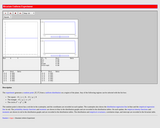Unrestricted Use
CC BY
Rating
0.0 stars

This resource consists of a Java applet and expository text. The Java applet illustrates the bivariate uniform distribution on three types of regions: a square, a circle, and a triangle. Simulated points from the distribution are shown as dots in a scatterplot.

Subject:
Mathematics
Statistics and Probability
Material Type:
Activity/Lab
Interactive
Simulation
Provider:
University of Alabama in Huntsville
Provider Set:
Virtual Laboratories in Probability and Statistics
Author:
Kyle Siegrist
02/16/2011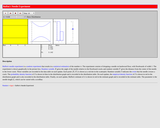Unrestricted Use
CC BY
Rating
0.0 stars

This resource consists of a Java applet and expository text. The applet simulates Buffon's needle experiment and the corresponding approximation of pi. The event of interest is that the needle crosses a crack. The length of the needle can be varied. The applet illustrates a random experiment, the sample space, random variables, probability, and relative frequency.

Subject:
Mathematics
Statistics and Probability
Material Type:
Activity/Lab
Interactive
Simulation
Provider:
University of Alabama in Huntsville
Provider Set:
Virtual Laboratories in Probability and Statistics
Author:
Kyle Siegrist
02/16/2011Conditional Remix & Share Permitted
CC BY-NC-SA
Rating
0.0 stars

This video module presents an introduction to cryptography - the method of sending messages in such a way that only the intended recipients can understand them. In this very interactive lesson, students will build three different devices for cryptography and will learn how to encrypt and decrypt messages. There are no prerequisites for this lesson, and it has intentionally been designed in a way that can be adapted to many audiences. It is fully appropriate in a high school level math or computer science class where the teacher can use it to motivate probability/statistics or programming exercises. nteractive lesson, students will learn to build the cryptography devices and will learn how to send and ''crack'' secret messages.

Subject:
Applied Science
Computer Science
Material Type:
Lecture
Provider:
MIT
Provider Set:
MIT Blossoms
Author:
Daniel J. Sturtevant